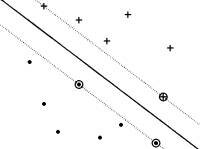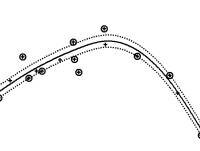HomeSupport Vector Machines SVM ReviewsSVM BooksSVM SoftwarePattern RecognitionOptimum HyperplaneSVM Regressionν-SVMSVM StatisticsMachine Learning MLnetNEuroNetEvoNetUCI RepositoryList 1List 2List 3WikipediaRepositoryROCKITWekaC4.5YALETutorialsSVM - Support Vector Machines
 Software
SVMlight
http://svmlight.joachims.org/
SVMlight, by Joachims, is one of the most widely used SVM classification and regression package. It has a fast optimization algorithm, can be applied to very large datasets, and has a very efficient implementation of the leave-one-out cross-validation. Distributed as C++ source and binaries for Linux, Windows, Cygwin, and Solaris. Kernels: polynomial, radial basis function, and neural (tanh).

SVMstruct
http://svmlight.joachims.org/svm_struct.html
SVMstruct, by Joachims, is an SVM implementation that can model complex (multivariate) output data y, such as trees, sequences, or sets. These complex output SVM models can be applied to natural language parsing, sequence alignment in protein homology detection, and Markov models for part-of-speech tagging. Several implementations exist: SVMmulticlass, for multi-class classification; SVMcfg, learns a weighted context free grammar from examples; SVMalign, learns to align protein sequences from training alignments; SVMhmm, learns a Markov model from examples. These modules have straightforward applications in bioinformatics, but one can imagine significant implementations for cheminformatics, when the chemical structure is represented as trees or sequences.

mySVM
http://www-ai.cs.uni-dortmund.de/SOFTWARE/MYSVM/index.html
mySVM, by Stefan Rüping, is a C++ implementation of SVM classification and regression. Available as C++ source code and Windows binaries. Kernels: linear, polynomial, radial basis function, neural (tanh), anova.

JmySVM
http://www-ai.cs.uni-dortmund.de/SOFTWARE/YALE/index.html
JmySVM, a Java version of mySVM is part of the YaLE (Yet Another Learning Environment) learning environment.

mySVM/db
http://www-ai.cs.uni-dortmund.de/SOFTWARE/MYSVMDB/index.html
mySVM/db is an efficient extension of mySVM which is designed to run directly inside a relational database using an internal JAVA engine. It was tested with an Oracle database, but with small modifications it should also run on any database offering a JDBC interface. It is especially useful for large datasets available as relational databases.

LIBSVM
http://www.csie.ntu.edu.tw/~cjlin/libsvm/
LIBSVM (Library for Support Vector Machines), is developed by Chang and Lin and contains C-classification, ν-classification, ε-regression, and ν-regression. Developed in C++ and Java, it supports also multi-class classification, weighted SVM for unbalanced data, cross-validation and automatic model selection. It has interfaces for Python, R, Splus, MATLAB, Perl, Ruby, and LabVIEW. Kernels: linear, polynomial, radial basis function, and neural (tanh).

looms
http://www.csie.ntu.edu.tw/~cjlin/looms/
looms, by Lee and Lin, is a very efficient leave-one-out model selection for SVM two-class classification. While LOO cross-validation is usually too time consuming to be performed for large datasets, looms implements numerical procedures that make LOO accessible. Given a range of parameters, looms automatically returns the parameter and model with the best LOO statistics. Available as C source code and Windows binaries.

BSVM
http://www.csie.ntu.edu.tw/~cjlin/bsvm/
BSVM, authored by of Hsu and Lin, provides two implementations of multi-class classification, together with SVM regression. Available as source code for UNIX/Linux and as binaries for Windows.

SVMTorch
http://www.idiap.ch/learning/SVMTorch.html
SVMTorch, by Collobert and Bengio, is part of the Torch machine learning library and implements SVM classification and regression. Distributed as C++ source code or binaries for Linux and Solaris.

Weka
http://www.cs.waikato.ac.nz/ml/weka/
Weka is a collection of machine learning algorithms for data mining tasks. The algorithms can either be applied directly to a dataset or called from a Java code. Contains an SVM implementation.

SVM in R
http://cran.r-project.org/src/contrib/Descriptions/e1071.html
This SVM implementation in R (http://www.r-project.org/) contains C-classification, n-classification, e-regression, and n-regression. Kernels: linear, polynomial, radial basis, neural (tanh).

M-SVM
http://www.loria.fr/~guermeur/
Multi-class SVM implementation in C by Guermeur.

Gist
http://microarray.cpmc.columbia.edu/gist/
Gist is a C implementation of support vector machine classification and kernel principal components analysis. The SVM part of Gist is available as an interactive web server at http://svm.sdsc.edu and it is a very convenient option for users that want to experiment with small datasets (several hundreds patterns). Kernels: linear, polynomial, radial.

MATLAB SVM Toolbox
http://www.isis.ecs.soton.ac.uk/resources/svminfo/
This SVM MATLAB toolbox, by Gunn, implements SVM classification and regression with various kernels: linear, polynomial, Gaussian radial basis function, exponential radial basis function, neural (tanh), Fourier series, spline, and B spline.

TinySVM
http://chasen.org/~taku/software/TinySVM/
TinySVM is a C++ implementation of C-classification and C-regression which uses sparse vector representation and can handle several ten-thousands of training examples, and hundred-thousands of feature dimensions. Distributed as binary/source for Linux and binary for Windows.

SmartLab
http://www.smartlab.dibe.unige.it/
SmartLab provides several support vector machines implementations: cSVM, Windows and Linux implementation of two-classes classification; mcSVM, Windows and Linux implementation of multi-classes classification; rSVM, Windows and Linux implementation of regression; javaSVM1 and javaSVM2, Java applets for SVM classification.

Gini-SVM
http://bach.ece.jhu.edu/svm/ginisvm/
Gini-SVM, by Chakrabartty and Cauwenberghs, is a multi-class probability regression engine that generates conditional probability distribution as a solution. Available as source code.

GPDT
http://dm.unife.it/gpdt/
GPDT, by Serafini, Zanni, and Zanghirati, is a C++ implementation for large-scale SVM classification in both scalar and distributed memory parallel environments. Available as C++ source code and Windows binaries.

HeroSvm
http://www.cenparmi.concordia.ca/~people/jdong/HeroSvm.html
HeroSvm, by Dong, is developed in C++, implements SVM classification, and is distributed as a dynamic link library for Windows. Kernels: linear, polynomial, radial basis function.

Spider
http://www.kyb.tuebingen.mpg.de/bs/people/spider/
Spider is an object orientated environment for machine learning in MATLAB, for unsupervised, supervised or semi-supervised machine learning problems, and includes training, testing, model selection, cross-validation, and statistical tests. Implements SVM multi-class classification and regression.

Java applets
http://svm.dcs.rhbnc.ac.uk/
These SVM classification and SVM regression Java applets were developed by members of Royal Holloway, University of London and AT&T Speech and Image Processing Services Research Lab.

LEARNSC
MATLAB scripts for the book Learning and Soft Computing by Kecman, implementing SVM classification and regression.

Tree Kernels
http://ai-nlp.info.uniroma2.it/moschitti/Tree-Kernel.htm
Tree Kernels, by Moschitti, is an extension of SVMlight, obtained by encoding tree kernels. Available as binaries for Windows, Linux, Mac-OSx, and Solaris. Tree kernels are suitable for encoding chemical structures, and thus this package brings significant capabilities for cheminformatics applications.

LS-SVMlab
http://www.esat.kuleuven.ac.be/sista/lssvmlab/
LS-SVMlab, by Suykens, is a MATLAB implementation of least squares support vector machines (LS-SVM) which reformulates the standard SVM leading to solving linear KKT systems. LS-SVM alike primal-dual formulations have been given to kernel PCA, kernel CCA and kernel PLS, thereby extending the class of primal-dual kernel machines. Links between kernel versions of classical pattern recognition algorithms such as kernel Fisher discriminant analysis and extensions to unsupervised learning, recurrent networks and control are available.

MATLAB SVM Toolbox
http://www.igi.tugraz.at/aschwaig/software.html
This is a MATLAB SVM classification implementation which can handle 1-norm and 2-norm SVM (linear or quadratic loss functions).

SVM/LOO
http://bach.ece.jhu.edu/pub/gert/svm/incremental/
SVM/LOO, by Cauwenberghs, has a very efficient MATLAB implementation of the leave-one-out cross-validation.

SVMsequel
http://www.isi.edu/~hdaume/SVMsequel/
SVMsequel, by Daume III, is a SVM multi-class classification package, distributed as C source or binaries for Linux or Solaris. Kernels: linear, polynomial, radial basis function, sigmoid, string, tree, information diffusion on discrete manifolds.

LSVM
http://www.cs.wisc.edu/dmi/lsvm/
LSVM (Lagrangian Support Vector Machine) is a very fast SVM implementation in MATLAB by Mangasarian and Musicant. It can classify datasets with several millions patterns.

ASVM
http://www.cs.wisc.edu/dmi/asvm/
ASVM (Active Support Vector Machine) is a very fast linear SVM script for MATLAB, by Musicant and Mangasarian, developed for large datasets.

PSVM
http://www.cs.wisc.edu/dmi/svm/psvm/
PSVM (Proximal Support Vector Machine) is a MATLAB script by Fung and Mangasarian which classifies patterns by assigning them to the closest of two parallel planes.

OSU SVM Classifier Matlab Toolbox
http://www.ece.osu.edu/~maj/osu_svm/
This MATLAB toolbox is based on LIBSVM.

SimpleSVM Toolbox
http://asi.insa-rouen.fr/~gloosli/simpleSVM.html
SimpleSVM Toolbox is a MATLAB implementation of the SimpleSVM algorithm.

SVM Toolbox
http://asi.insa-rouen.fr/%7Earakotom/toolbox/index
A fairly complex MATLAB toolbox, containing many algorithms: classification using linear and quadratic penalization, multi-class classification, ε-regression, ν-regression, wavelet kernel, SVM feature selection.

MATLAB SVM Toolbox
http://theoval.sys.uea.ac.uk/~gcc/svm/toolbox/
Developed by Cawley, has standard SVM features, together with multi-class classification and leave-one-out cross-validation.

R-SVM
http://www.biostat.harvard.edu/~xzhang/R-SVM/R-SVM.html
R-SVM, by Zhang and Wong, is based on SVMTorch and is specially designed for the classification of microarray gene expression data. R-SVM uses SVM for classification and for selecting a subset of relevant genes according to their relative contribution in the classification. This process is done recursively in such a way that a series of gene subsets and classification models can be obtained in a recursive manner, at different levels of gene selection. The performance of the classification can be evaluated either on an independent test data set or by cross-validation on the same data set. Distributed as Linux binary.

jSVM
jSVM is a Java wrapper for SVMlight.

SvmFu
http://five-percent-nation.mit.edu/SvmFu/
SvmFu, by Rifkin, is a C++ package for SVM classification. Kernels: linear, polynomial, and Gaussian radial basis function.

PyML
http://pyml.sourceforge.net/
PyML is an interactive object oriented framework for machine learning in Python. It contains a wrapper for LIBSVM, and procedures for optimizing a classifier: multi-class methods, descriptor selection, model selection, jury of classifiers, cross-validation, ROC curves.

BioJava
http://www.biojava.org/
BioJava is an open-source project dedicated to providing a Java framework for processing biological data. It include objects for manipulating sequences, file parsers, DAS client and server suport, access to BioSQL and Ensembl databases, and powerful analysis and statistical routines including a dynamic programming toolkit. The package org.biojava.stats.svm contains SVM classification and regression.

 Search "SVM" in: PubMedPubMed CentralCiteSeerGoogle ScholarGoogleScirusBioChem PressSearch "Support Vector" in: PubMedPubMed CentralCiteSeerGoogle ScholarGoogleScirusBioChem PressJournals JMLRIEJMDBioinformaticsNucleic Acids ResearchBioMed CentralLiterature Databases PubMedPubMed CentralCiteSeerSearch Engines DOAJScirusOJOSE